# pointers parameters and const

Note: All Examples are written in C++

# Pointers

Pointers are “variables” that do not hold actual content but the address where the content can be found.

We first should make sure that we know the underlying technologie. Whenever we declare a variable/object, this requires some memory in which the content is stored. We can think of the memory as a table, we store our content in the rows of the table where each row has a unique address.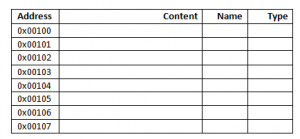So lets assume that we now declare a variable

`int test=4;`

Then (usually) the Operating system decides where to store this variable. Lets assume it is stored at 0x00101 then our memory would look like this: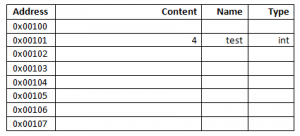whenever we now use the variable, it is known where to find the corresponding value.

We need to introduce 2 operators:

##### Reference operator (&)

The reference operator is giving us back the address of a variable.

`&test;`

would therefore give us 0x00101 as this is the address of “test”.

##### Dereference operator (*)

The dereference operator works the other direction of the reference operator. If we give have stored an address then we can use the dereference operator to access the content. That is the basic mechanism of pointers.

Lets assume we want to declare a pointer to an integer. We use an asterisk (*) to tell the compiler that this is not an integer but a pointer to an integer:

`int* p_test;`

Its good coding practice to name the pointers with the prefix “p_” (or similar) so its clear to everyone that this is a pointer. Of course this pointer will also require some memory by itself as the address it is holding need to be saved somewhere.

Right now there is no content, the only thing we did so far is that we declared the pointer, no integer has been declared so far! Our memory could like like this: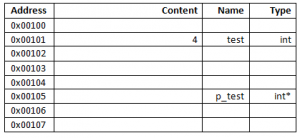We now want to make our pointer to point to the integer “test” we have defined before:

`p_test=&test;`

which means that we get the adress of “test” (0x00101) and save it to “p_test”.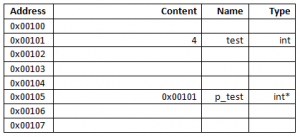We now have a complete pointer. Its obvious that if we access p_test like a regular variable, we get back the the address (the Content) of it but not the value of the integer we pointed to.

`p_test;`

is giving us 0x00101.

If we want to access the content of the value we are pointing to we now need to use the dereference operator:

`*p_test:`

is giving us “4” as we look up the content at 0x00101.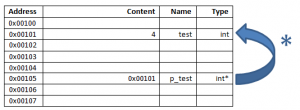Also we can use the dereference operator to get the address of “p_test”

`&p_test;`

is giving us 0x00105 as this is the address where the pointer is stored.

From the memory above we can now create the following tables: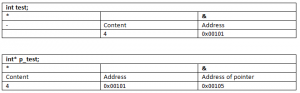##### Example
```// Header
#include <iostream>		// Header for std IO (e.g. cout, cin)
#include <stdlib.h>

using namespace std;	// with this we do not need to write std::cout, we can directly use cout

int main (void)
{
// declare an integer
int normalinteger;

// we now have allocated memory for our "normalinteger", but we can not control what had been stored in this memory before
// therefore we need to initialize the variable with some sensible content
normalinteger=1;

//now we can have a look at the value as well as the address
cout<<"An integer, the value: "<<normalinteger<<" and the Address: "<<&normalinteger<<endl;

// now we declare an pointer to an integer
int* p_integer;

// as there was the need to initialize "normalinteger", there is also the need to initialize this pointer.
// in this example we want the pointer to point to the "normalinteger".

//get the Adress of "normalinteger" and save it to "p_integer"
p_integer=&normalinteger;

//lets have a look at p_integer now
cout<<"A Pointer, dereference it: "<<*p_integer<<" the value: "<<p_integer<<" and the dereferencing (the adress of the pointer itself): "<<&p_integer<<endl;

// you can see that if we want to access the content we are pointing to, we need the dereference operator
// lets assign a value

*p_integer=5;

// as we have pointed to "normalinteger" we now can see that we changed the value of it
cout<<"The integer after we have assigned a value via pointer: "<<normalinteger;

return 0;
}```
##### Pointers to pointers

We can use pointers to other pointers:

```	// declare and initialize the final target
int normalinteger=5;

// the first pointer
int* pointer_to_integer=&normalinteger;

//the second pointer, note the double asterisk
int** pointer_to_integer_pointer=&pointer_to_integer;

//we now can access the value using the double asterisk
cout<<"The value of the actual integer:"<<**pointer_to_integer_pointer<<endl;```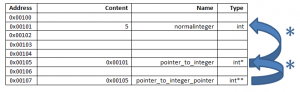So first we dereference “pointer_to_integer_pointer” which is giving us the content of “pointer_to_integer” which is the address to “normalinteger”, so we dereference this address again to get the actual value.

##### “new” and “delete”

So far we always have declared variables which are directly coupled to a variable name, like

`int myint=1337;`

.

There is also the possibility to instantiate variables/objects which are not coupled to a variable name. All we get is an address in the memory. We then can use a pointer to access this variable/object. We do so by using the “new” keyword.

```//set up a pointer to an int
int* p_to_int;
//create a new integer. "new" is giving back the address of the new integer which is then stored to the pointer.
p_to_int = new int(4);```

It is very important to delete this variable/object after we have used it to make sure the memory is freed so it can be used otherwise.

`delete *p_to_int;`

Please note that the address of the deleted object is still stored within the pointer and it might be possible to still use it. BUT the memory is not allocated to us anymore, so it can change at any time which can lead to big catastrophes. Therefore very often the pointer is assigned to “null” to indicate that it is not in use at the moment. However, the memory allocated is freed if the program ends, therefore its up to you to decide whether its usefull to free the memory.

##### Pointers and Arrays

Pointers can be used to control arrays. After we have declared an array, we can use a pointer to point to the first address of the array. We then can use increment and decrement operators to navigate through the array.

```    // declare an array of integers
int arrayofintegers;

//declare an pointer to an integer (because our array is an array of integers)
int* p_integer;

//we now set the pointer to the starting address of the array
p_integer=arrayofintegers;

//lets fill the array with some content
for(unsigned i=0; i<5;i++)
arrayofintegers[i]=i;

//we check the content and the addresses of the array elements
for(unsigned i=0; i<5;i++)
cout<<"Array at Position "<< i << " has value: "<<arrayofintegers[i] <<" and is at address " << &arrayofintegers[i]<<endl;

//As you can see the addresses of the elements are ascending

cout<<endl<<endl;

//we set our pointer to the first address of the array ( "arrayofintegers" gives us the same value as &arrayofintegers)
p_integer=arrayofintegers;

//as we have declared an pointer to an integer, it is known how much the pointer has to increment to reach the next element, +1 will not work
for(unsigned i=0; i<5;i++)
{
cout<<"Array at Position "<< i << " has value: "<<*p_integer <<" and is at address " << p_integer<<endl;
p_integer++;
}

cout<<endl<<endl;

//we even can get further
for(int* i=arrayofintegers; i!=&arrayofintegers;i++)
{
cout<<"Array at address "<< i << " has value: "<<*i <<endl;
}
```

As we have seen above we can use pointers to point to elements within an array. Also the array can be filled with pointers:

```	// declare and initialize the final target
int* array_of_pointers;

// pointer to our value, note that this is a double pointer, because we point to an array (first *) of pointers (second *)
int** p_pointer_to_integer=array_of_pointers;

//Fill the array using just the pointer
for(unsigned i=0;i<5;i++)
{
//we need to use "new" here as we need to allocate memory
*p_pointer_to_integer=new int(i);
p_pointer_to_integer++;
}

for(unsigned i=0;i<5;i++)
cout<<*array_of_pointers[i]<<endl;

for(int** i=array_of_pointers;i!=&array_of_pointers;i++)
cout<<**i<<endl;
```

The memory in the example could look like this if we place “p_pointer_to_integer” to the first element in the array.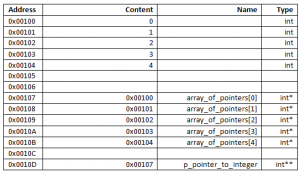# parameter passing

There are different ways to pass parameters to a function/method.

### Call by value

If we pass parameters by call by value we copy the parameter to a inner variable, this has the benefit that the variable which is used within the function/method is an own copy of the parameter, on the other hand we can not use this variable to pass content out of the function/method and also we need more memory to realize this.

```void call_by_value(int var1, double var2){
var1=42;
}

int main (void)
{
int 	m_var1=10;
double 	m_var2=20;

call_by_value(m_var1,m_var2);
}```

In the example above the values of “m_var1” and “m_var2” are copied to “var1” and “var2”.
“var1” and “var2” then can be used inside “call_by_value”.

### Call by pointer

If we call by pointer then we will give the address(es) where the parameters can be found to the function/method. We now can write directly to the given parameters (to m_var1,m_var2) from within the function/method. This has the benefit that we do not occupy additional memory and do not need to copy the values, but on the other hand, if there is something going wrong within the function/method, the original values might be lost. Note that we first need to dereference the pointer to access its content.

```void call_by_pointer(int* var1, double* var2){
*var1=42;
}

int main (void)
{
int 	m_var1=10;
double 	m_var2=20;

call_by_pointer(&m_var1,&m_var2);
}```

### Call by reference

This approach is very similar to call by pointer but has one difference, we do not need to dereference when assigning a value and we do not need to get the address when calling the function/method.

```void call_by_reference(int & var1, double & var2){
var1=42;
}

int main (void)
{
int 	m_var1=10;
double 	m_var2=20;

call_by_reference(m_var1,m_var2);
}```

The problem here is that it is not obvious at the first sight that we are dealing with a reference and not with an own value. To avoid misunderstandings we can use “const”.

# Const

“Const” can be used to disallow a value to be overwritten.
For normal variables its quite easy:

`const double pi=3.1415926535897932384626433832795028841971693993751058209749445923078164;`

will disallow to overwrite “pi” with another value.

For Parameter Passing with call by reference or pointer its a bit more complicated. Here it depends on the position. Is it left/right of */& or on both sides.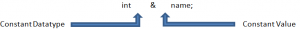If the Datatype is constant the dereferenced value can not be changed.
If the Value (aka the Address) is constant, it can not be changed, so we can not point to another instance.

If you use “const” for the return value of a function/method then the return value is changeable in its content but not in its address.

This site uses cookies. By continuing to browse the site you are agreeing to our use of cookies.